# Smart Energy Monitor

🔌 Load Monitoring and Energy Disaggregation on a RasPi
Alternatives To Smart Energy Monitor
Deepc448
a year ago26apache-2.0C++
vendor independent TinyML deep learning library, compiler and inference framework microcomputers and micro-controllers
Eloquentarduino132
a year ago7C++
IO, scheduling, utils, machine learning... for Arduino
Colabs95
2 months agootherJupyter Notebook
This repository holds the Google Colabs for the EdX TinyML Specialization
Gestures Ml Js61
4 years ago2gpl-3.0JavaScript
[WIP] - Gesture recognition using hardware and Tensorflow.js
Rgb Neural Net60
6 years agogpl-3.0Jupyter Notebook
Physical visualisation of neural network learning using RGB leds, arduino and raspberry pi.
Esp32_cloudspeech40
5 years ago1mitC++
Machine Learning For Physical Computing37
2 years agoJavaScript
Repository for the "Machine Learning for Physical Computing" class at ITP, NYU
Aisonobuoy30
a month ago6apache-2.0C
Maritime Situational Awareness: An Exploration
Arduino Library27
10 months ago1otherC++
This repository holds the Arduino Library for the EdX TinyML Specialization
Esp32 Autonomous Car25
a year agomitPython
Autonomous car using ESP32.
Alternatives To Smart Energy Monitor
Select To Compare

Alternative Project ComparisonsThe goal of the Smart Energy Monitor is to accurately predict the monthly electricity bill of the household using minimum hardware & by acquiring electrical data at a single location (instead of individual sensors per appliance). We do this by analyzing the current & power signatures of all the active devices and pass this information through a Naive Bayes classifier which helps us obtain the Active devices which can further be used to calculate the number of units consumed by individual load appliances.

## Features

• Detect when a particular appliance has changed its state (On/Off/Other).
• Classify the active load appliances by analyzing the measured electrical signals which have been obtained from a single location.
• Tracking of individual appliance-level consumption.
• Electricity bill prediction.
• Hardware used to obtain current, active and reactive power signatures of individual devices to create high quality datasets.

## Prerequisites

Hardware:

1. Raspberry Pi running Raspbian
2. Arduino Nano
3. Current & Voltage Transformers
4. Additional Electronic components required for the Zero Cross Detection circuit.

Software:

1. Flask - Web App Microframework
2. Dash by Plotly - Analytical Web App Framework (Optional)

## Working

Combinatorial Algorithm (CA):
The combinatorial algorithm is a brute-force method to determine the active appliances. CA finds the optimal combination of appliance states, which minimizes the difference between the sum of the predicted appliance power and the observed aggregate power, subject to a set of appliance models. The complexity of disaggregation for T time slices is: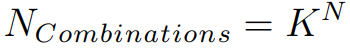where N is the number of appliances and K is the number of appliance states. Since the complexity of CA is exponential to the number of appliances, the approach is only computationally tractable for a small number of modelled appliances. Hence, we chose to use a Naive Bayes classifier to determine the active appliances. A toy demonstration example is used to visualize how the number of computations increases as the number of appliances increases.

Power Measurement:
We can measure peak current by sampling the mains power line until we obtain the maximum and minimum peak voltage values (i.e. the maximum value measured in each direction). We can then compute the RMS voltage value from the peak-to-peak voltage. For the ACS712 we can convert the RMS voltage value into an RMS current value using the scale factor given in the datasheet . We can then measure the power being consumed using the voltage and current RMS values.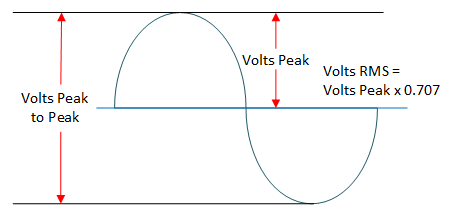Steps to find Power for a sine wave with a zero volt offset:

1. Find the Peak to Peak voltage (Vpp) of the ACS712 current sensor.
2. Divide Vpp by 2 to get the peak voltage in one direction.
3. Multiply the peak voltage by 0.707 to get the RMS voltage for the ACS712.
4. Convert the RMS voltage into RMS current by multiplying by the scale factor given for the particular ACS712 model.
5. Multiply the measured voltage with the RMS current to find the power being drawn by the loads.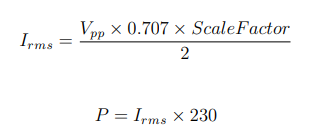Real power and reactive power are two of the most commonly used steady state signatures in NILM for tracking On/Off operation of appliances. The real power is the amount of energy consumed by an appliance during its operation. If the load is purely resistive then the current and voltage waveforms will always be in phase and there will be no reactive energy. For a purely reactive load the phase shift will be 90 degrees, and there will be no transfer of real power. On the other hand, due to inductive and capacitive elements of the load, there is always a phase shift between current and voltage waveforms that generates or consumes a reactive power respectively.

Monitoring Changes in Current and Power:
The current drawn by the load is the first parameter used to classify the various load appliances. The current sensor will provide us with the instantaneous value of the total current drawn by all loads connected to the Smart Energy Monitor. In order to get the value of the current drawn by each individual load appliance we must calculate the difference between each of the consecutive current sample.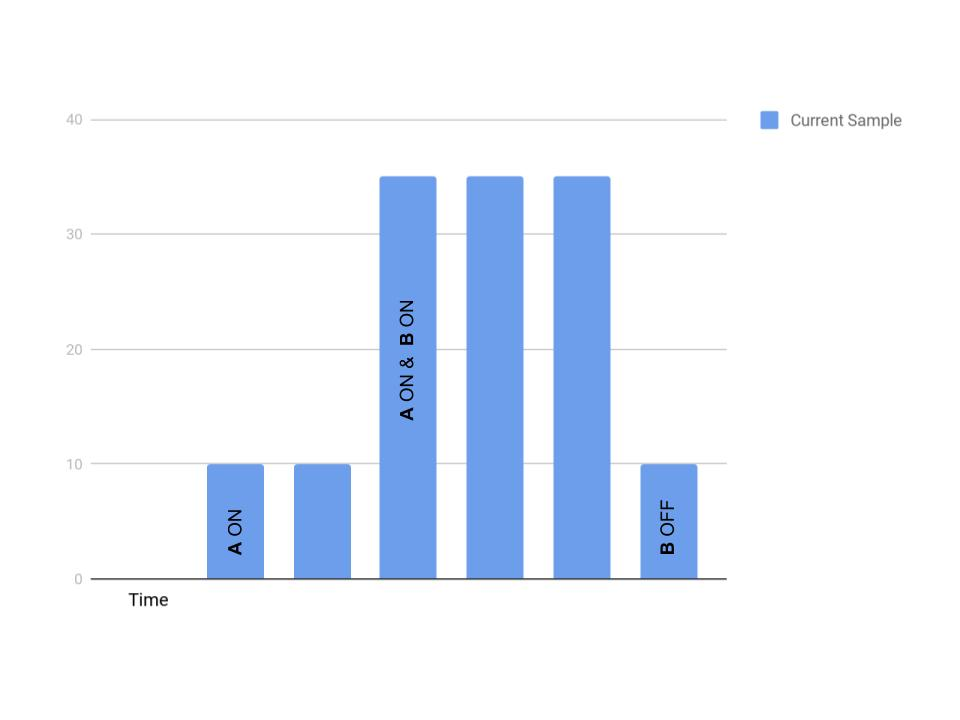As shown in the above figure, we can see that when load appliance A is turned ON, the change in current is 10, when B is turned ON after A, the change in current is 25 but the total current is 35 and finally when B is turned OFF the change in current is 25 while the total current drawn is 10.

The total change in current that occurs when a load appliance is switched ON/OFF may not be correctly reflected between the two consecutive samples. Instead the total change in current may be reflected over more than two consecutive current samples depending on the switching speed or transient time of the load appliances. Hence, occasionally large errors are produced when measuring the current difference between any two consecutive current samples. In order to reduce the error, the sampling rate may be adjusted accordingly. But calibrating the sampling rate may be difficult as various load appliances have different transient times as well as the time taken for different software instructions may differ with different hardware and sensors.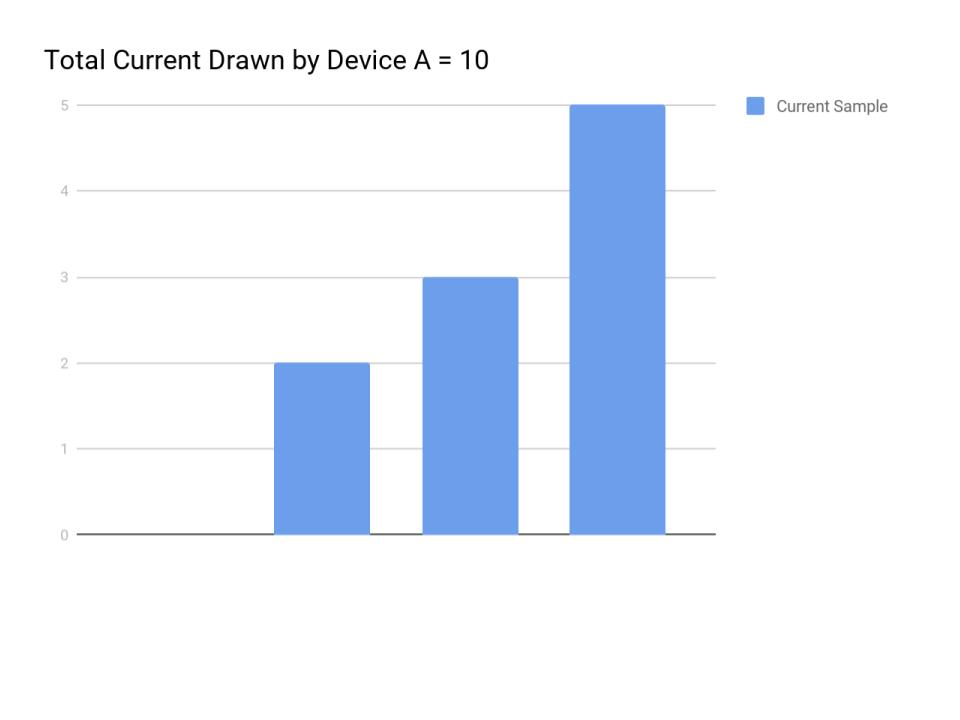A more efficient method for reducing this error will be to find the sum of N consecutive current samples such that the sum of all the error is zero(or close to zero). The user may have to wait for a very short duration before the next load appliance can be switched ON/OFF. The small error that remains will not come into effect as the classification algorithm will rule it out based on the mean and standard deviation values of all the labelled data. As shown in the above figure, load appliance A during its transient state as a total current change of 10. But this value is not reflected between any two consecutive current samples. Instead the change in current observed between any two consecutive current samples for load appliance A is 2,3 and 5 respectively. Hence the value of error produced will be either 3 or 5. But if we take the sum of N consecutive samples where in N in this case is equal to 3, then we will get an error value of 0.

Active and Reactive Power:
Using only the current drawn by a load as a classification parameter will cause problems when two or more completely different load appliances (with different applications) draw the same amount of current. This is because, the current difference between N consecutive samples for both the devices may be equal which may produce an incorrect result.

Hence in order to more accurately differentiate between load appliances which draw the same amount of current, we also consider the value of active power and reactive power drawn by each individual load appliance. It will be highly unlikely that two completely different load appliances with different applications have the same current drawn as well as active power and reactive power values.In order to calculate the Active Power and Reactive Power drawn by load appliances, we must first find the phase difference between the voltage and current. We do this by implementing a simple Zero Cross Detector (ZCD) for both the voltage and current.

The ZCD is built using the LM339. The amplitude of the measured current and voltage signals is reduced to meet the maximum input value permitted by the LM339. A screenshot of the phase angle measurement circuit is shown.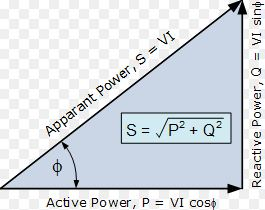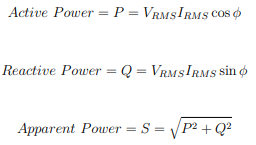The output of the ZCD is fed to a single Ex-OR gate of a 7486 EXOR IC which will produce pulses when there is a phase shift. i.e. The two logic levels of the inputs to the EXOR gate are not equal to each other.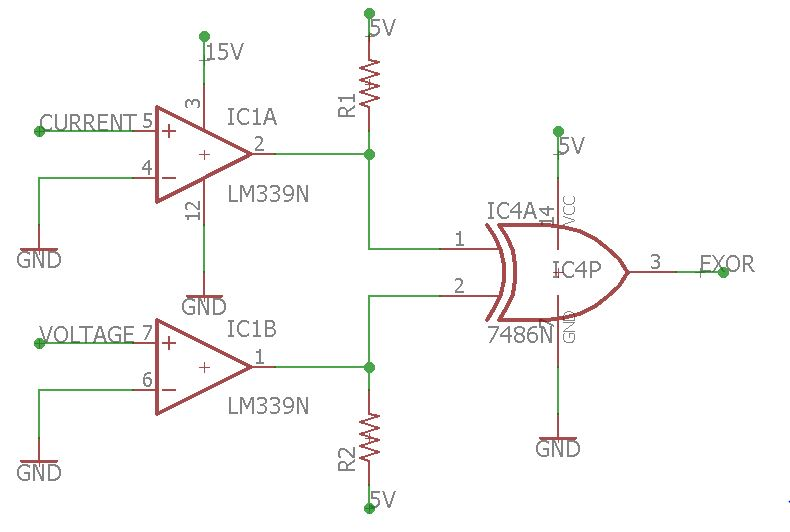The time period of these pulses can be used to find the phase angle between the voltage and current waveforms.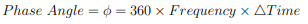Creating the Dataset:
Each appliance will have at least two class labels associated with it depending upon the number of 'Activity States' it may undergo during normal operation. Typically most appliances will have only two states. E.g: A light bulb will have only two states - On and Off. Whereas, a kitchen mixer may have several states depending on the adjustable mixer mode. Each state is defined by its separate current and power attributes. Each sample is a measurement of the change in either current, active power or reactive power with the ground truth label being one of the appliance activity states.

Determining the Appliance State: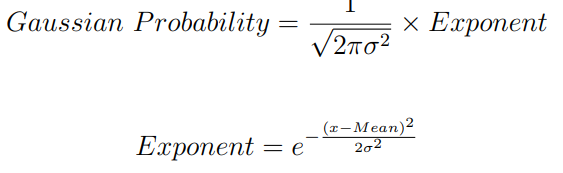We use a Naive Bayes classification algorithm to determine which appliances are active and in which activity state are they operating in. Predictions are made by choosing the class with the closest mean and standard deviation for each feature when compared with the training data using a Gaussian Probability Density function. i.e We combine the probability of each feature to determine the class probability.

In the Demo Example we use a Demo training dataset which contains the measured features for 3 devices with a total of 6 states namely two CFL bulbs and an electric drill.

You can find the video of this Demo Example: Demo Video

We can use additional features such as Weather, Time and Room to improve the prediction accuracy.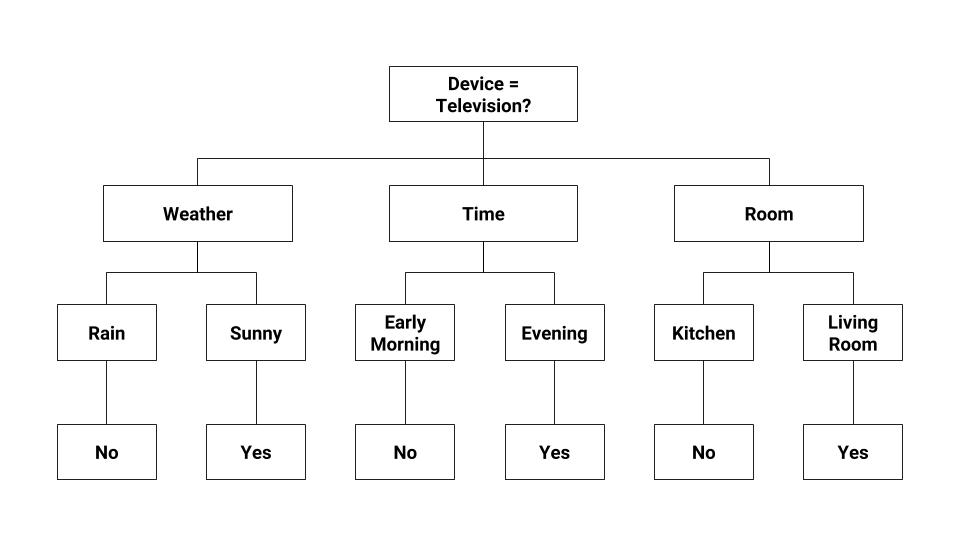These additional features can be used as features within a decision tree to be used as an ensemble together with the existing Naive Bayes classifier.

Estimating Electricity Bill:
Once we have determined the active devices. We can use measure the total power they individually consume by measuring their Start and Stop time (Total Active Time = Start Time - Stop Time) and simply multiplying the power consumed by the appliance for each activity state by the total active time for a particular state. We can then estimate the monthly electricity bill by multiplying the power consumed over the entire month by the rate per unit. An example of a blank table for the Demo Example is shown below.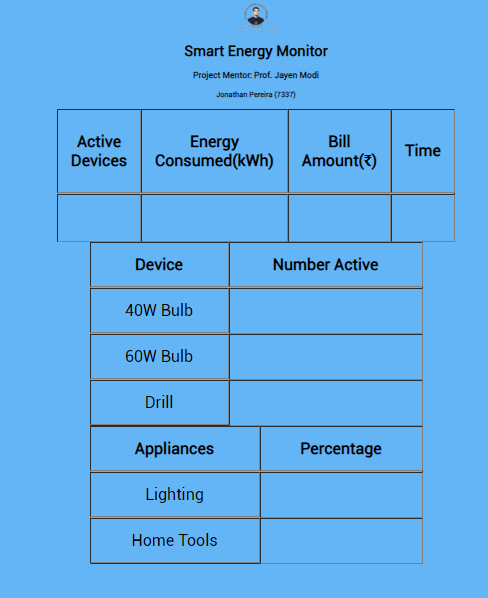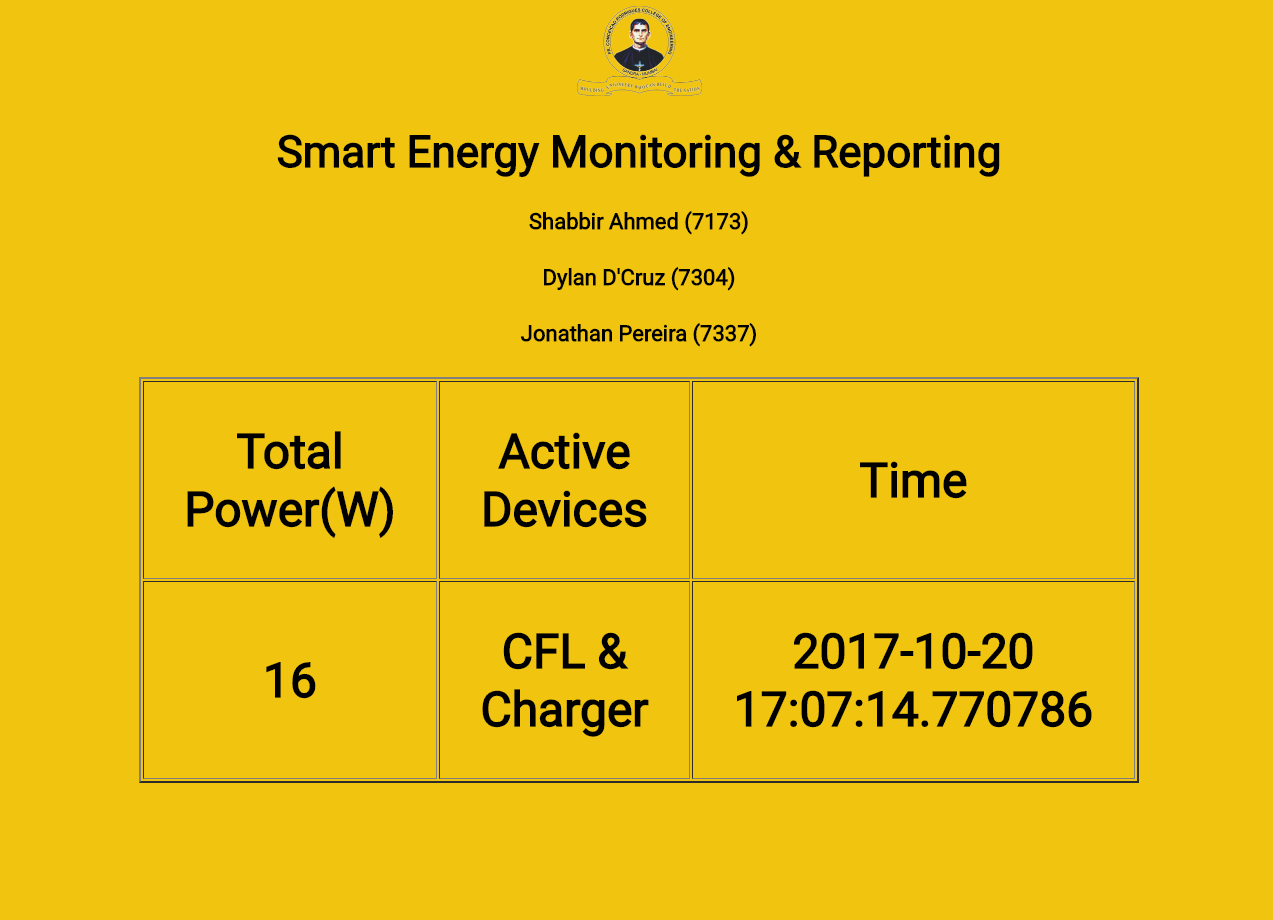User Interface:
The user interface uses a Python Micro-Framework called Flask which is used to build web applications. The Flask framework encodes the real time Python Variables into a JavaScript Object Notation (JSON) string. The JSON string is then read into the HTML file using Asynchronous JavaScript And XML (AJAX) requests. These requests are periodically refreshed using the Auto-Refresh function in AJAX. Basic UI Auto Data Generator Demo uses auto-updating date/time information as dummy data sent to the HTML Dashboard Demo.

## Future Work

• The classification accuracy can be improved through pattern analysis by monitoring the shape of the VI trajectory. Shape features such as area under the VI curve as well as peak of segments can be further analyzed. 
• Analysis of Steady State Voltage Noise such as EMI signatures can improve the detection of motor based devices like fans, food mixers and washing machines. Although this would require additional EMI sensors for each appliance which will be contradiction with the initial goal of this project.
• Using Hidden Markov Models  and Neural Networks  to determine active devices.
• Graphs for energy consumed per month per appliance.

## Contributing

Are you a programmer, engineer or hobbyist who has a great idea for a new feature in this project? Maybe you have a good idea for a bug fix? Feel free to grab our code from Github and tinker with it. Don't forget to smash those ⭐️ & Pull Request buttons. Contributor List

Made with ❤️ by Jonathan Pereira

## References

Popular Machine Learning Projects
Popular Arduino Projects
Popular Machine Learning Categories
Related Searches

Get A Weekly Email With Trending Projects For These Categories
No Spam. Unsubscribe easily at any time.
Python
Machine Learning
Arduino
Raspberry Pi
Electronics
Smart Home